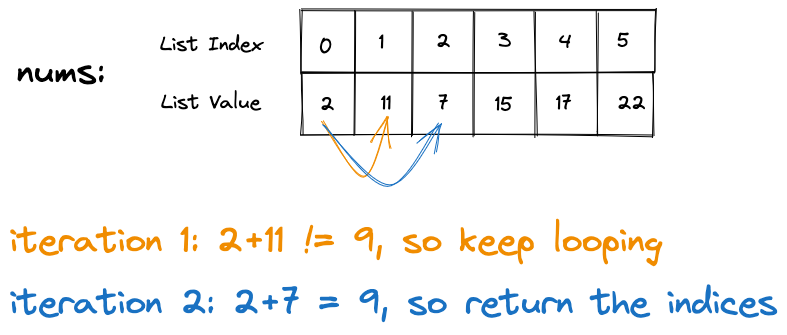# LeetCode: Two Sum

`10 minute read`

## Prompt

Given an array of integers nums and an integer target, return indices of the two numbers such that they add up to target.
You may assume that each input would have exactly one solution, and you may not use the same element twice.
You can return the answer in any order.

## What are the inputs and outputs?

• Inputs:
• array of integers `nums`
• integer `target`
• Output:
• array of integers

## Brute Force Solution:

For each index:

• iterate through each subsequent index
• if the sum of the values of the indices equal the target
• then return the respective indices```def twoSum(nums, target):
"""
:type nums: List[int]
:type target: int
:rtype: List[int]
"""
for idx1, num1 in enumerate(nums):
for idx2, num2 in enumerate(nums):
if idx1 == idx2:
continue
if num1 + num2 == target:
return [idx1, idx2]
def test_twoSum():
test_nums = [2,11,7,15,17,22]
test_target = 9
expected_result = [0,2]
result = twoSum(test_nums, test_target)
print(expected_result == result)
test_twoSum()
```

While this is a solution to the problem, we would run into issues if the target was 39 (the sum of the last 2 values in the list).
For all the indices, we would have to go through each other index.
In our particular example, our list is size 6 so 6x6 or 62 would be 36.
We can generalize this to say that for any list size n our worst case would be n2.
We can do better!

## Optimized Solution

In the optimized solution, we create a dictionary with the key being the value of each index we iterate through and the value being the index.
We can now use the key to determine if we can use stored index for each subsequent index.

Pseudo code:
For each item in our list:

• get the difference between the target and the value
• if the difference is already a key in our seen dictionary, then we can return that index along with the current one
• else add the current value as a new key to the dictionary with the current index as the value
```def twoSum(nums, target):
"""
:type nums: List[int]
:type target: int
:rtype: List[int]
"""

seen = {}

for index, value in enumerate(nums):
diff = target - value
if diff in seen:
return [seen[diff], index]
else:
seen[value] = index

def test_twoSum():
test_nums = [2,11,7,15,17,22]
test_target = 39
expected_result = [4,5]
result = twoSum(test_nums, test_target)
print(expected_result == result)
test_twoSum()

```

In our first iteration, our dictionary would be `seen: {"2": 0}`
After 5 iterations, our dictionary would be `seen: {"2": 0, "11": 1, "7": 2, "15": 3, "17": 4 }`
On our 6th iteration, we are looking for a key of the target (39) - the value of the current index (22) = 17 which we do find!
We then return the value of the dictionary for the key we found along with the current index.